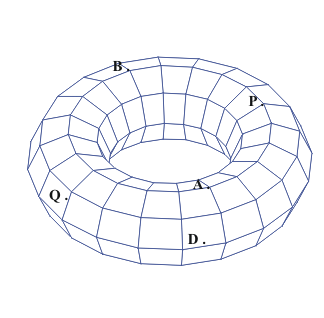Thursday,  April  20, 2006
More on Areas, Rates, and Slopes

Recall from last class:

Arithmetic growth:  2, 7, 12, 17, 22, ...
Examples?
Formula: A (x)  = 5x + 2;  Y(x) = mx + b,  m>0
Other examples using approximations- measurements of curves and surfaces:
Archimedes: The area of the circle- the area of a triangle.
Kepler: The volume of a torus- the volume of a cylinder.Growth Rates: m - linear, r - exponential.
Graphing and rates: slopes of lines, approximating curves with lines.
Note: The simplest non-linear algebraic curves are the conics: especially- the parabolas.
slope of straight line:
m =  (change in y)/(change in x)
Problem: Find the Area "under a straight line":
Motion: time: t ; position:s
constant and average velocity:
v = (change in s)/(change in t)
Problem: Given a constant velocity or a constant acceleration for a moving object, find the distance travelled.
Note: The motion of a falling object is perhaps the simplest physical example of motion with a variable velocity. Such an object has a constant acceleration.
Questions:
What is the "slope" on a curved line?
What is the instaneous velocity of a falling object?
What is the area of a region in the plane bounded by a curved line?
Given an object moving at a variable velocity, how far will it travel in a fixed interval of  time?

Activity: Estimations of slopes of tangent lines and area for a parabola given by a quadratic relation between coordinates y and x.

"zooming"
What if motion does not proceed at constant velocity?

Example: What line does the graph of  y = x2 look like near (3,9).

Related measurement concepts:
• Instantaneous Rate of growth or motion.
• Slope (gradient) of a curved line, tangent line to a curve.
• Net Change in measured variable based on rate of growth.
• Area of a region in the plane.

What is the calculus?
A systematic method for answering these questions of velocity, tangents, change in position and area that uses the algebraic representation of a relation between y and x .
Examples: if y = .... then the slope of the tangent to the curve described by this relationship at the point (a,b) is....
If the position s = ... t.... then the instantaneous velocity of the object moving to corresponfd to this relation  at time t is .................
If the velocity of a moving object is given by v= .... t..... , then the object has a net change during the interval from t = a to t = b of .....
the area of the region in the plane bounded by the Xis, the lines X = a and X= b and the graph of y= ...x... is....